Category: Data Structures

A Doubly Linked List is a linear data structure which collectively represents a sequence of data in which each node has three parts. In this tutorial, we will learn about the implementation of a doubly linked list and various operations on it like insertion, deletion and traversal of a Doubly Linked List and it's advantages and disadvantages over Singly Linked List.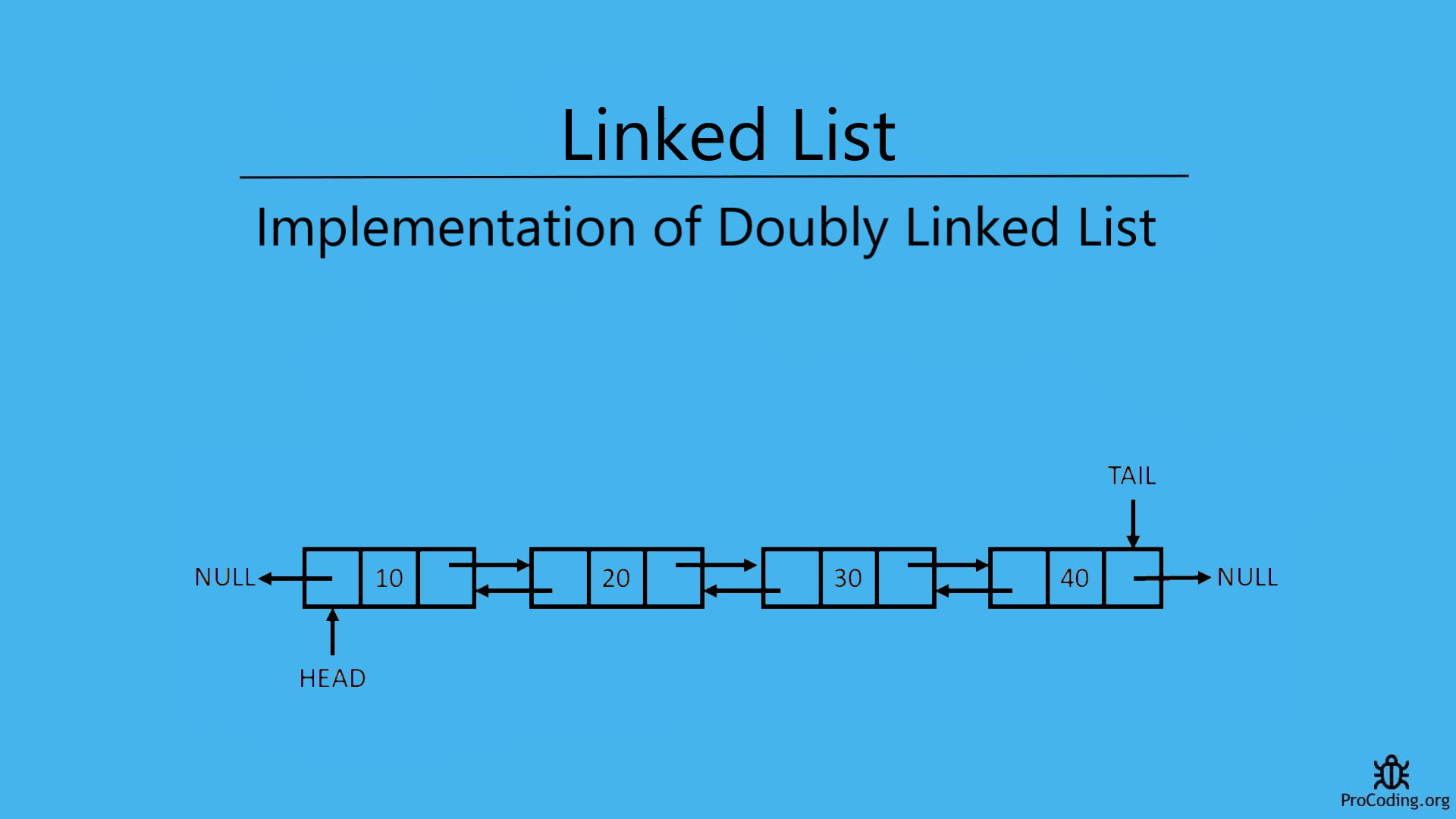A Doubly Linked List is a collection of nodes in which each node has three parts i.e, `pointer to previous node`, `data` and `pointer to next node` and last node points to `null`.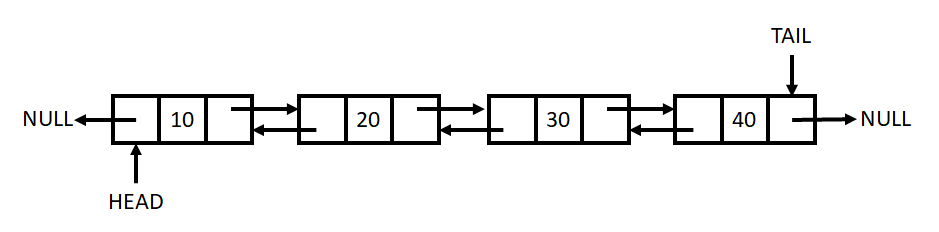## Declaration of type node for Doubly Linked List

We have to create a data structure or Node which contains three part i.e, first part contains address of previous node, second part contains data of the node and third node contains address of next pointer. We can create this by using user defined data type (depends on the programming language used).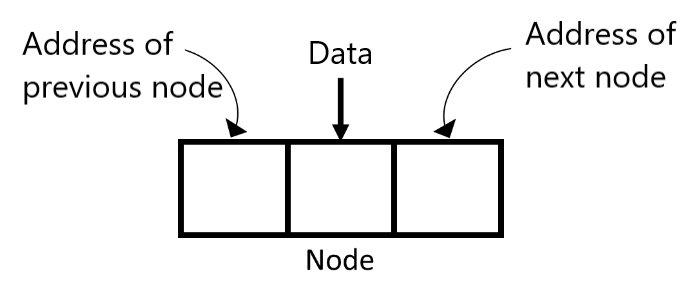Python
``````class Node:
def __init__(self, data):
self.prev = None
self.data = data
self.next = None
``````
JavaScript
``````class Node {
constructor(data) {
this.prev = null;
this.data = data;
this.next = null;
}
}
``````

## Traversing the Doubly Linked List

Traversing means visiting all the node once i.e, we want to read all the data available in the doubly linked list. We can do so by visiting all the nodes one by one and reading the data available in each node.

Simply, use a temporary variable and initialize it with the head node so that we can start with the begining of the list and then go through all the nodes one by one till the last node is reached. And we can get the last node by checking the next value of node i.e., if next value of node is `null` then this is the end of node.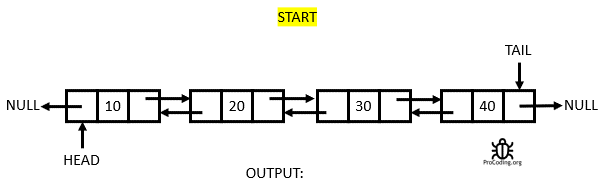Python
``````def traverse(self):
while temp:
print(temp.data)
temp = temp.next
else:
print(None)
``````
JavaScript
``````traverse() {
while (temp) {
console.log(temp.data);
temp = temp.next;
}
} else {
console.log(null);
}
}
``````

## Reverse traversing of the Doubly Linked List

Reverse traversing means visiting all the node once from the backward direction i.e, we want to read all the data available in the doubly linked list from the tail. We can do so by visiting all the nodes one by one and reading the data available in each node.

Simply, use a temporary variable and initialize it with the tail node so that we can start with the end of the list and then go through all the nodes one by one till the first node is reached. And we can get the first node by checking the next value of node i.e., if next value of node is `null` then this is the begining of node.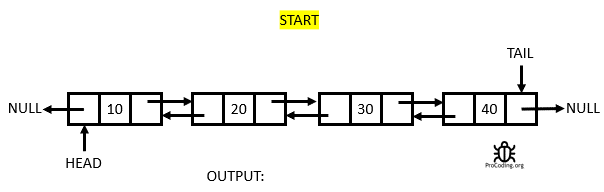Python
``````def reverse_traverse(self):
if self.tail:
temp = self.tail
while temp:
print(temp.data)
temp = temp.prev
else:
print(None)
``````
JavaScript
``````reverseTraverse() {
if (this.tail) {
let temp = this.tail;
while (temp) {
console.log(temp.data);
temp = temp.prev;
}
} else {
console.log(null);
}
}
``````

## Length of a Doubly Linked List

To find length of a linked list we can simply use a temporary variable and initialize it by assigning the head node. Now, move it to every node one by one and increase the counter variable.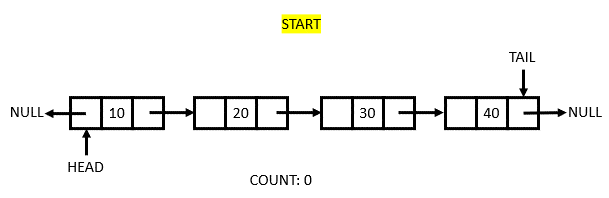Python
``````def length(self):
count = 0
while temp:
count += 1
temp = temp.next
return count
``````
JavaScript
``````length() {
let count = 0;
while (temp) {
count++;
temp = temp.next;
}
return count;
}
``````

## Insertion in a Doubly Linked List

Insertion in a Linked List can be done in following three ways:

### Insertion at the begining of the Doubly Linked List

While inserting a node at the begining position, two situation may arise:

1. Insertion when Doubly Linked List is emtpy: In this case we can simply create a new node and put the value in data part, null into the next part and previous part (which is already done in Class Node constructor) then assign it to the head.
2. Insertion when Doubly Linked List is not empty: In this case create a new node, add value into the data part and assign the node pointed by the head (current starting node) to next of newly created node and then head will now point to new node and null into the previous part (which is already done in Class Node constructor).Python
``````def insert_at_start(self, data):
new_node = Node(data)

self.tail = new_node
else:
``````
JavaScript
``````insertAtStart(data) {
const newNode = new Node(data);

this.tail = newNode;
} else {
}
}
``````

### Insertion at the end of the Doubly Linked List

While inserting a node at the end position, two situation may arise:

1. Insertion when Doubly Linked List is emtpy: In this case we can simply create a new node and put the value in data part, null into the next part and previous part (which is already done in Class Node constructor) then assign it to the head.
2. Insertion when Doubly Linked List is not empty: In this case create a new node, and then assign it to the next part of tail node then tail should point to the newly created node.Python
``````def insert_at_end(self, data):
new_node = Node(data)

self.tail = new_node
else:
new_node.prev = self.tail
self.tail.next = new_node
self.tail = new_node
``````
JavaScript
``````insertAtEnd(data) {
const newNode = new Node(data);

this.tail = newNode;
} else {
newNode.prev = this.tail;
this.tail.next = newNode;
this.tail = newNode;
}
}
``````

### Insertion at given position in the Doubly Linked List

While inserting at any given position following 3 situation may arise based on user input:

1. Insertion at the begining: In this case, simply call the function used to insert at begining.
2. Insertion at the end: In this case, simply call the function used to insert at the end.
3. Insertion at any random position: In this case, use a temporary variable and initialize with the head pointer traverse till the `given position - 1` and then insert the new node by adjusting the previous and next pointer.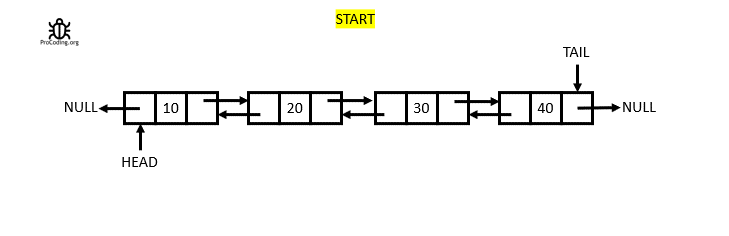Python
``````def insert_at_position(self, pos, data):
new_node = Node(data)

if pos < 0 or pos > self.length():
raise Exception('Enter a valid postion')
else:
if pos == 0:
self.insert_at_start(data)
elif pos == self.length()-1:
self.insert_at_end(data)
else:
for _ in range(pos-1):
temp = temp.next
new_node.next = temp.next
temp.next = new_node
new_node.prev = temp
``````
JavaScript
``````insertAtPosition(pos, data) {
const newNode = new Node(data);

if (pos < 0 || pos > this.length()) {
throw "Enter a valid position";
} else {
if (pos == 0) {
this.insertAtStart(data);
} else if (pos == this.length() - 1) {
this.insertAtEnd(data);
} else {
for (let i = 0; i < pos - 1; i++) {
temp = temp.next;
}
newNode.next = temp.next;
temp.next = newNode;
newNode.prev = temp;
}
}
}
``````

## Deletion in a Doubly Linked List

Same as insertion deletion in a Linked List can be done in 3 ways:

### Deletion from the begining of the Doubly Linked List

While deleting a node at the from begining, two situation may arise:

• Deletion when Linked List is emtpy: When Linked List is empty throw an error that Linked List is empty.
• Deletion when Linked List is not empty: Again, two cases may arise if linked list is not empty
1. If only one node is present: In this case, delete that node and set head and tail as `null`
2. If multiple nodes are present: Then shift the head pointer to next node and set `null` to the new previous part of new head node.

For languages like Python, Java etc. which have in built mechanism for garbage collection, can remove the deleted node itself but in case of languages like C or C++ we have to point that node and remove it manually.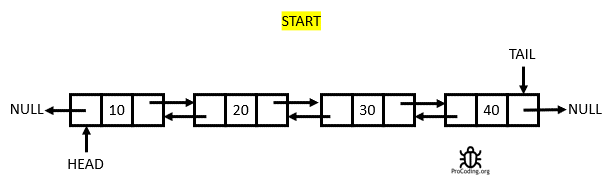Python
``````def delete_from_start(self):
else:
return data
else:
``````
JavaScript
``````deleteFromStart() {
let data;
} else {
}
return data;
} else {
}
}
``````

### Deletion from the end of the Doubly Linked List

While deleting a node at the from the end, two situation may arise:

• Deletion when Linked List is emtpy: Again two cases may arise if linked list is not empty
• Deletion when Linked List is not empty: Again two cases may arise if linked list is not empty
1. If only one node is present: In this case, delete that node and set head and tail as `null`
2. If multiple nodes are present: Then shift the tail node to the previous node and set `null` to the next part of new tail node.

For languages like Python, Java etc. which have in built mechanism for garbage collection, can remove the deleted node itself but in case of languages like C or C++ we have to point that node and remove it manually.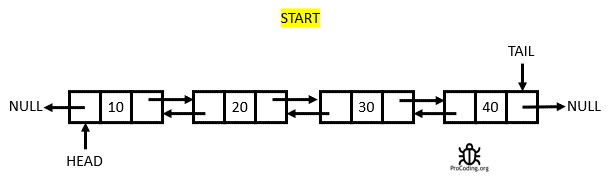Python
``````def delete_from_end(self):
else:
data = self.tail.data
self.tail = self.tail.prev
self.tail.next = None
return data
else:
``````
JavaScript
``````deleteFromEnd() {
let data;
} else {
data = this.tail.data;
this.tail = this.tail.prev;
this.tail.next = null;
}
return data;
} else {
}
}
``````

### Deletion from given position in the Doubly Linked List

While deleting at any given position following 3 situation may arise based on user input:

1. Deletion from the begining: In this case, simply call the function used to delete from begining.
2. Deletion from the end: In this case, simply call the function used to delete from the end.
3. Deletion from any random position: In this case, use a temporary variable and initialize with the head pointer and traverse till the given node is reached by the given position and adjust the next and previous pointers of the nodes situated after and before this node. For languages like Python, Java etc. which have in built mechanism for garbage collection, can remove the deleted node itself but in case of languages like C or C++ we have to point that node and remove it manually.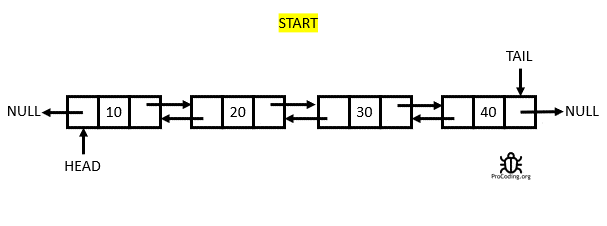Python
``````def delete_at_position(self, pos):
if pos < 0 or pos >= self.length():
raise Exception('Enter a valid postion')
else:
if pos == 0:
return self.delete_from_start()
elif pos == self.length()-1:
return self.delete_from_end()
else:
for _ in range(pos):
temp = temp.next
data = temp.data
prev_node = temp.prev
prev_node.next = temp.next
temp.next.prev = prev_node
return data
``````
JavaScript
``````deleteAtPosition(pos) {
if (pos < 0 || pos >= this.length()) {
throw "Enter a valid position";
} else {
if (pos == 0) {
return this.deleteFromStart();
} else if (pos === this.length() - 1) {
return this.deleteFromEnd();
} else {
for (let i = 0; i < pos; i++) {
temp = temp.next;
}
const data = temp.data;
const prevNode = temp.prev;
prevNode.next = temp.next;
temp.next.prev = prevNode;
return data;
}
}
}
``````

## Implementation of Doubly Linked List

Implementation of singly linked list linked list with all the function combined with output.

Python
``````class Node:
def __init__(self, data):
self.prev = None
self.data = data
self.next = None

def __init__(self):
self.tail = None

def insert_at_start(self, data):
new_node = Node(data)

self.tail = new_node
else:

def insert_at_end(self, data):
new_node = Node(data)

self.tail = new_node
else:
new_node.prev = self.tail
self.tail.next = new_node
self.tail = new_node

def insert_at_position(self, pos, data):
new_node = Node(data)

if pos < 0 or pos > self.length():
raise Exception('Enter a valid postion')
else:
if pos == 0:
self.insert_at_start(data)
elif pos == self.length()-1:
self.insert_at_end(data)
else:
for _ in range(pos-1):
temp = temp.next
new_node.next = temp.next
temp.next = new_node
new_node.prev = temp

def delete_from_start(self):
else:
return data
else:

def delete_from_end(self):
else:
data = self.tail.data
self.tail = self.tail.prev
self.tail.next = None
return data
else:

def delete_at_position(self, pos):
if pos < 0 or pos >= self.length():
raise Exception('Enter a valid postion')
else:
if pos == 0:
return self.delete_from_start()
elif pos == self.length()-1:
return self.delete_from_end()
else:
for _ in range(pos):
temp = temp.next
data = temp.data
prev_node = temp.prev
prev_node.next = temp.next
temp.next.prev = prev_node
return data

def get_first(self):
else:

def get_last(self):
if self.tail:
return self.tail.data
else:

def traverse(self):
while temp:
print(temp.data)
temp = temp.next
else:
print(None)

def reverse_traverse(self):
if self.tail:
temp = self.tail
while temp:
print(temp.data)
temp = temp.prev
else:
print(None)

def length(self):
count = 0
while temp:
count += 1
temp = temp.next
return count

dll.insert_at_end(1)
dll.insert_at_end(2)
dll.insert_at_end(3)
dll.insert_at_end(4)
dll.insert_at_end(5)
dll.insert_at_end(6)
dll.traverse()
``````
JavaScript
``````class Node {
constructor(data) {
this.prev = null;
this.data = data;
this.next = null;
}
}

constructor() {
this.tail = null;
}

insertAtStart(data) {
const newNode = new Node(data);

this.tail = newNode;
} else {
}
}

insertAtEnd(data) {
const newNode = new Node(data);

this.tail = newNode;
} else {
newNode.prev = this.tail;
this.tail.next = newNode;
this.tail = newNode;
}
}

insertAtPosition(pos, data) {
const newNode = new Node(data);

if (pos < 0 || pos > this.length()) {
throw "Enter a valid position";
} else {
if (pos == 0) {
this.insertAtStart(data);
} else if (pos == this.length() - 1) {
this.insertAtEnd(data);
} else {
for (let i = 0; i < pos - 1; i++) {
temp = temp.next;
}
newNode.next = temp.next;
temp.next = newNode;
newNode.prev = temp;
}
}
}

deleteFromStart() {
let data;
} else {
}
return data;
} else {
}
}

deleteFromEnd() {
let data;
} else {
data = this.tail.data;
this.tail = this.tail.prev;
this.tail.next = null;
}
return data;
} else {
}
}

deleteAtPosition(pos) {
if (pos < 0 || pos >= this.length()) {
throw "Enter a valid position";
} else {
if (pos == 0) {
return this.deleteFromStart();
} else if (pos === this.length() - 1) {
return this.deleteFromEnd();
} else {
for (let i = 0; i < pos; i++) {
temp = temp.next;
}
const data = temp.data;
const prevNode = temp.prev;
prevNode.next = temp.next;
temp.next.prev = prevNode;
return data;
}
}
}

getFirst() {
}

getLast() {
if (this.tail) return this.tail.data;
}

traverse() {
while (temp) {
console.log(temp.data);
temp = temp.next;
}
} else {
console.log(null);
}
}

reverseTraverse() {
if (this.tail) {
let temp = this.tail;
while (temp) {
console.log(temp.data);
temp = temp.prev;
}
} else {
console.log(null);
}
}

length() {
let count = 0;
while (temp) {
count++;
temp = temp.next;
}
return count;
}
}

dll.insertAtEnd(1);
dll.insertAtEnd(2);
dll.insertAtEnd(3);
dll.insertAtEnd(4);
dll.insertAtEnd(5);
dll.insertAtEnd(6);
dll.traverse();
``````

Output

``````1
2
3
4
5
6
``````